# Getting Started with Plotly in R

• Last Updated : 06 Jun, 2021

Plotly in R Programming Language allows to create interactive web graphics from ‘ggplot2’ graphs and a custom interface to the JavaScript library ‘plotly.js’ inspired by the grammar of graphics.

### Installation

To use a package in R programming one must have to install the package first. This task can be done using the command install.packages(“packagename”). To install the whole plotly package type this:

install.packages(“plotly”)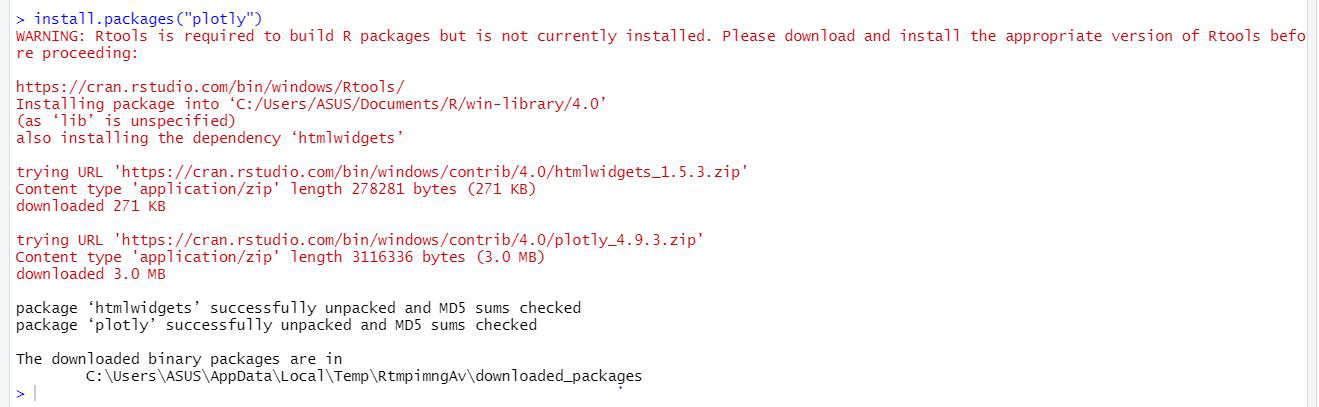Or install the latest development version (on GitHub) via devtools:

devtools::install_github(“ropensci/plotly”)

### Important Functions

• plot_ly: It basically initiates a plotly visualization. This function maps R objects to plotly.js, an (MIT licensed) web-based interactive charting library. It provides abstractions for doing common things and sets some different defaults to make the interface feel more ‘R-like’ (i.e., closer to plot() and ggplot2::qplot()).

Syntax:

plot_ly(data = data.frame(), …, type = NULL, name, color, colors = NULL, alpha = NULL, stroke, strokes = NULL, alpha_stroke = 1, size, sizes = c(10, 00), span, spans = c(1, 20), symbol, symbols = NULL, linetype, linetypes = NULL, split, frame, width = NULL, height = NULL, source = “A”)

Example:

## R

 `# import plotly library` `library``(plotly)`   `# create plotly visualisation` `p <- ``plot_ly``(iris, x = ~Sepal.Width, ` `                   ``y = ~Sepal.Length) `   `# adding markers` `add_markers``(p, color = ~Petal.Length,` `               ``size = ~Petal.Length)` `add_markers``(p, color = ~Species)`

Output: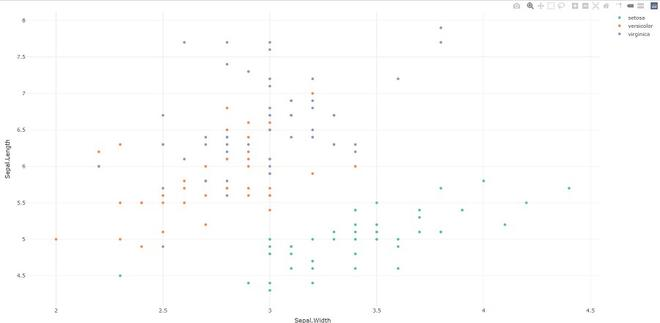• plotly_build: This generic function creates the list object sent to plotly.js for rendering. Using this function can be useful for overriding defaults or for debugging rendering errors.

Syntax: plotly_build(p, registerFrames = TRUE)

Example:

## R

 `# import plotly library` `library``(plotly)`   `# create plotly visualisation` `p <- ``plot_ly``(iris, x = ~Sepal.Width,` `                    ``y = ~Sepal.Length) `   `# structure of plotly using` `# plotly_build` `str``(``plotly_bulid``(p, registerFrames = ``TRUE``))`

Output:

No scatter mode specified:

Setting the mode to markers

List of 8

\$ x            :List of 10

..\$ visdat     :List of 1

.. ..\$ 29f4345ac49:function ()

..\$ cur_data   : chr “29f4345ac49”

..\$ attrs      :List of 1

.. ..\$ 29f4345ac49:List of 5

.. .. ..\$ x           :Class ‘formula’  language ~Sepal.Width

.. .. .. .. ..- attr(*, “.Environment”)=<environment: R_GlobalEnv>

.. .. ..\$ y           :Class ‘formula’  language ~Sepal.Length

.. .. .. .. ..- attr(*, “.Environment”)=<environment: R_GlobalEnv>

.. .. ..\$ alpha_stroke: num 1

.. .. ..\$ sizes       : num [1:2] 10 100

.. .. ..\$ spans       : num [1:2] 1 20

……..

• layout: Modify the layout of a plotly visualization

Syntax: layout(p, …, data = NULL)

Example:

## R

 `# import plotly library` `library``(plotly)`   `# create plotly visualisation` `p <- ``plot_ly``(iris, x = ~Sepal.Width,` `                    ``y = ~Sepal.Length) ` `layout``(p, data = ``NULL``)`

Output: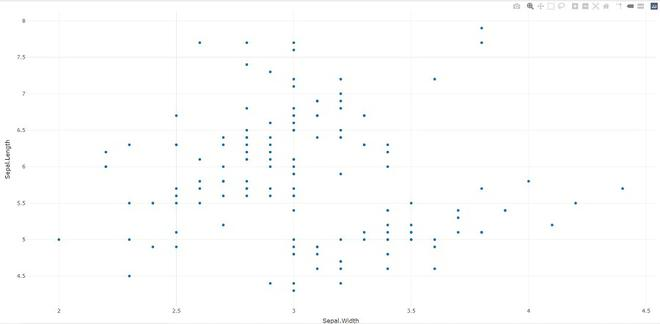Syntax: add_trace(p, …, data = NULL, inherit = TRUE)

Example:

## R

 `# import plotly library` `library``(plotly)`   `# create plotly visualisation` `p <- ``plot_ly``(iris, x = ~Sepal.Width, ` `                    ``y = ~Sepal.Length) `   `# adding trace (lines) to plotly` `# visualisation` `add_trace``(p, type = ``"scatter"``, ` `          ``mode = ``"markers+lines"``)`

Output: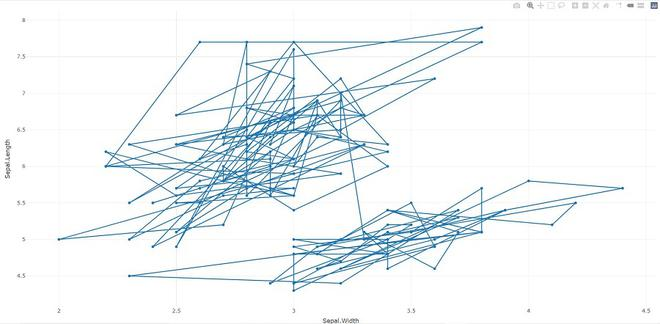• animation_opts: Provides animation configuration options. Animations can be created by either using the frame argument in plot_ly() or frame ggplot2 aesthetic in ggplotly(). By default, animations populate a play button and slider component for controlling the state of the animation (to pause an animation, click on a relevant location on the slider bar). Both the play button and slider component transition between frames according to rules specified by animation_opts().

Syntax:

animation_opts(p, frame = 500, transition = frame, easing = “linear”, redraw = TRUE, mode = “immediate”)

animation_slider(p, hide = FALSE, …)

animation_button(p, …, label)

Example:

## R

 `# import plotly library` `library``(plotly)`     `plot_ly``(mtcars, x = ~wt, y = ~mpg, ` `        ``frame = ~cyl) %>%` `animation_opts``(transition = 0)`

Output: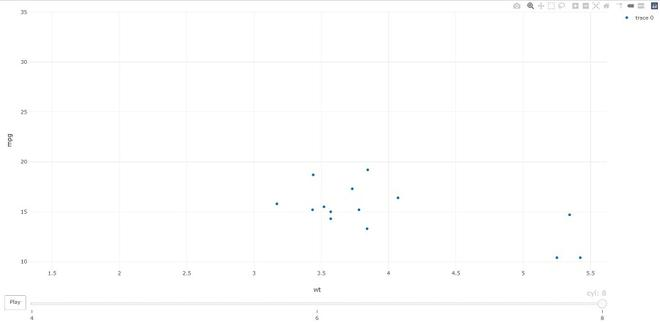Example:

## R

 `# import plotly library` `library``(plotly)`     `plot_ly``() %>% ``add_data``(economics) %>% ` `        ``add_trace``(x = ~date, y = ~pce)`

Output: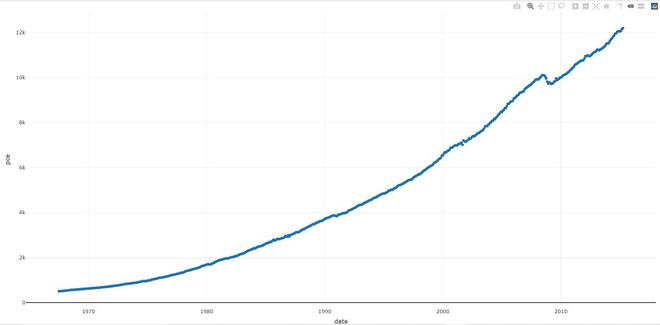• plotly_IMAGE: Creates a static image for plotly visualization. The images endpoint turns a plot (which may be given in multiple forms) into an image of the desired format.

Syntax:

plotly_IMAGE(x, width = 1000, height = 500, format = “png”, scale = 1, out_file, …)

Example:

## R

 `# import plotly library` `library``(plotly)`   `# create plotly visualisation` `p <- ``plot_ly``(iris, x = ~Sepal.Width,` `                    ``y = ~Sepal.Length)`   `# importing plotly visualisation ` `# as image files` `Png <- ``plotly_IMAGE``(p, ` `                    ``out_file = ``"plotly-test-image.png"``)` `Jpeg <- ``plotly_IMAGE``(p, format = ``"jpeg"``,` `                     ``out_file = ``"plotly-test-image.jpeg"``)`   `# importing plotly visualisation` `# as vector graphics` `Svg <- ``plotly_IMAGE``(p, format = ``"svg"``,  ` `                    ``out_file = ``"plotly-test-image.svg"``)`   `# importing plotly visualisation as` `# pdf file` `Pdf <- ``plotly_IMAGE``(p, format = ``"pdf"``, ` `                    ``out_file = ``"plotly-test-image.pdf"``)`

Output: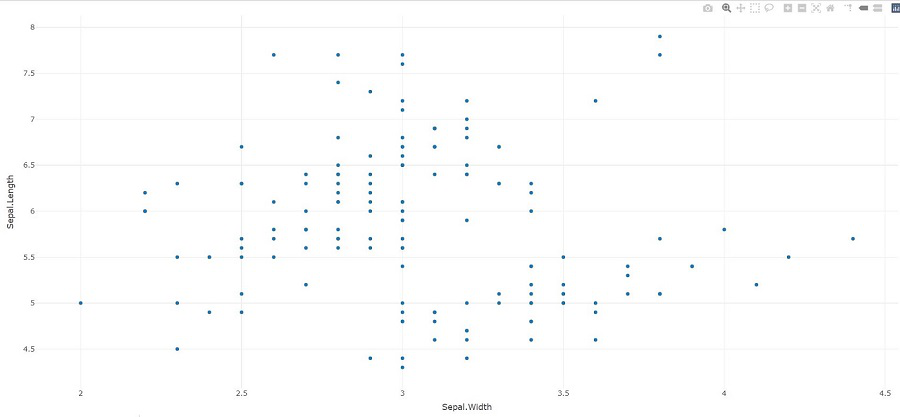• plotly_empty: Creates a complete empty plotly graph. It is a very useful function when used with subplot().

Syntax: plotly_empty(…)

Example:

## R

 `# import plotly library` `library``(plotly)` `plotly_example``(iris)`

Output: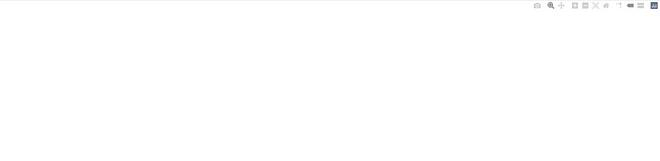• plotly_example: It runs a plotly example(s). Provides a unified interface for running demos, shiny apps, and Rmd documents which are bundled with the package.

Syntax: plotly_example(type = c(“demo”, “shiny”, “rmd”), name, edit = TRUE, …)

Example:

## R

 `# import plotly library` `library``(plotly)` `plotly_example``(type = ``c``(``"demo"``, ``"shiny"``, ``"rmd"``),` `               ``name, edit = ``TRUE``, ...)`

Output: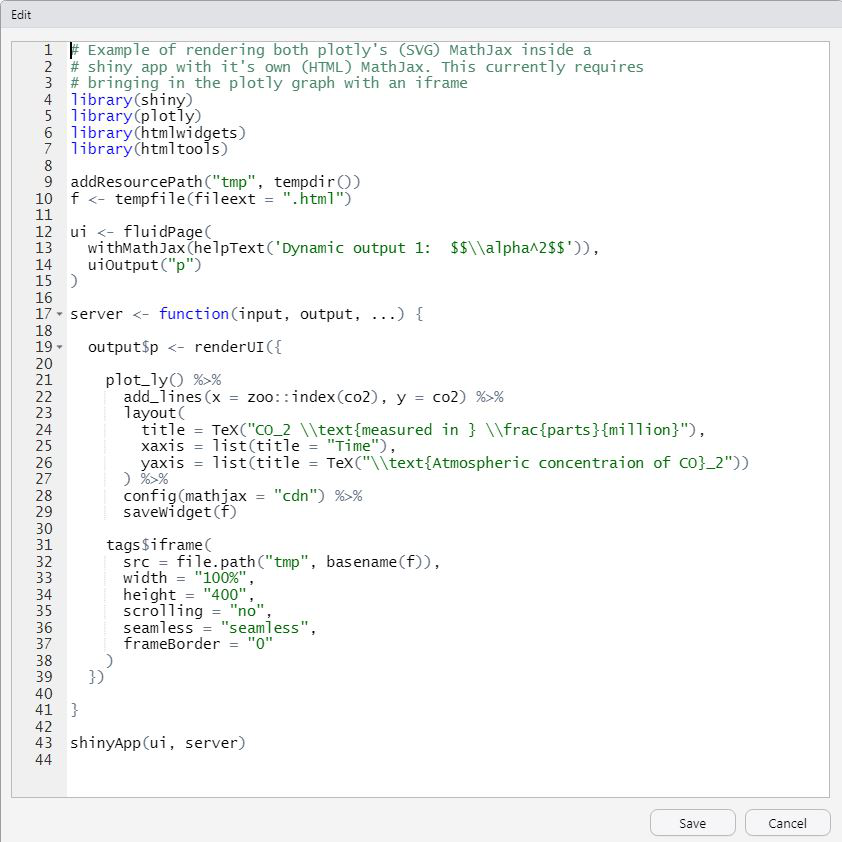We can leverage the plotly R package to create a variety of interactive graphics. Two main ways of creating a plotly object: either by transforming a ggplot2 object (via ggplotly()) into a plotly object or by directly initializing a plotly object with plot_ly()/plot_geo()/plot_mapbox(). Both approaches have somewhat complementary strengths and weaknesses, so it can pay off to learn both approaches.

My Personal Notes arrow_drop_up
Recommended Articles
Page :